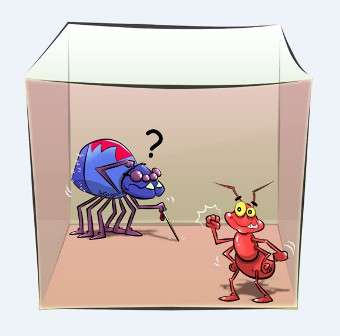Mathproblems.info
Formerly known as Shack's Math Problems
By Michael Shackleford

Welcome to my site of 236 and counting math problems. Anyone with an appreciation of a good math problem should find something they like here, with problems ranging from basic math to differential equations.

## The Math ProblemsLooking for a specific problem? Try the index.
math humor.

Other Web Pages of Mine